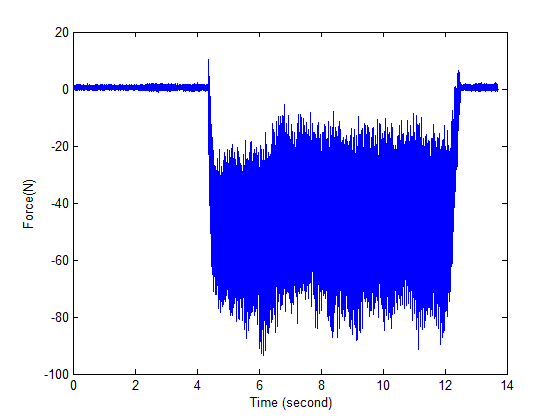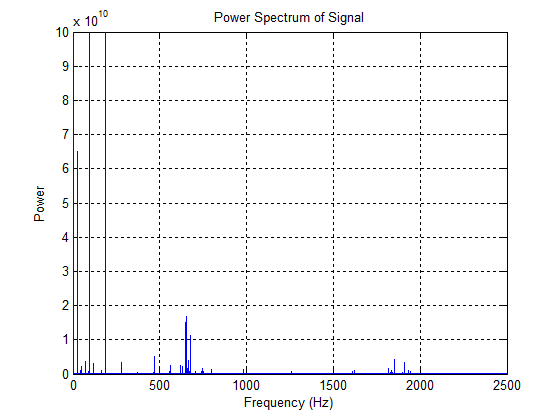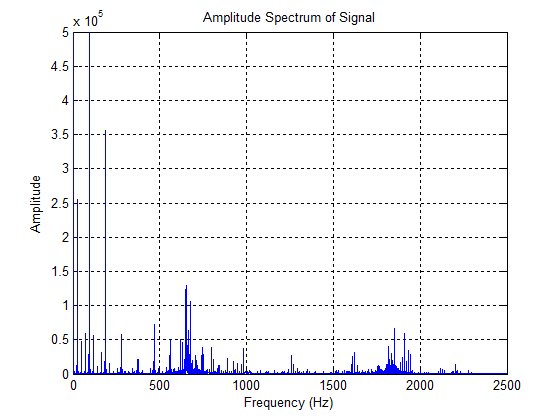# Signal Analysis

Hzaqa

## Homework Statement

Hi ,
The problem is how to analysis this signal in terms of time segments to understnd the characteristics/pattern of this signal## Homework Equations

There is no relevant equtions

## The Attempt at a Solution

I have tried to use :
RMS
Wavlet
FFT
But which one of them can give us a better understing of the system,

Thanks,

## The Attempt at a Solution

Homework Helper
Gold Member
Your problem statement is fairly unconstrained so--

For frequency analysis, consider making a spectrogram, a 3 dimensional graph (frequency vs amplitude vs time).

For amplitude analysis, experiment with various moving average (RMS) plots.

Mentor
Presumably you have a data set that corresponds to the graph, and not just the graph alone? You might want to investigate the use of the Lomb-Scargle Periodogram method to tease out the underlying frequency components of the signal.

Hzaqa
Thanks for the response,

Well, I have applied frequency domain

Matlab code:

m=fft(x)
n=length(x)
power=abs(m(1:floor(n/2))).^2
nyquist=1/2
freq=((1:n/2)/(n/2)*nyquist)*5000
figure
plot(freq,power)
xlabel('Frequency (Hz)')
ylabel('Power')
title('Power Spectrum of Signal')
grid onamp=abs(m(1:floor(n/2)))
figure
plot(freq,amp)
xlabel('Frequency (Hz)')
ylabel('Amplitude')
title('Amplitude Spectrum of Signal')
grid onBut I am not quite sure which one of them is best/correct,any suggestion,
Thanks